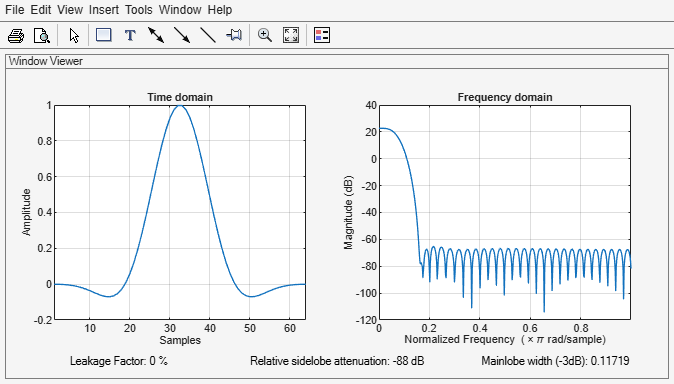Documentation

### This is machine translation

Mouseover text to see original. Click the button below to return to the English version of the page.

# flattopwin

Flat top weighted window

## Syntax

``w = flattopwin(L)``
``w = flattopwin(L,sflag)``

## Description

example

````w = flattopwin(L)` returns an `L`-point symmetric flat top window ```
````w = flattopwin(L,sflag)` an `L`-point symmetric flat top window using the window sampling method specified by `sflag`.```

## Examples

collapse all

Create a 64-point symmetric flat top window. View the result using `wvtool`.

```N = 64; w = flattopwin(N); wvtool(w)```## Input Arguments

collapse all

Window length, specified as a positive integer.

Data Types: `single` | `double`

Window sampling method, specified as:

• `'symmetric'` — Use this option when using windows for filter design.

• `'periodic'` — This option is useful for spectral analysis because it enables a windowed signal to have the perfect periodic extension implicit in the discrete Fourier transform. When `'periodic'` is specified, the function computes a window of length `L` + 1 and returns the first `L` points.

## Output Arguments

collapse all

Flat top window, returned as a column vector.

## Algorithms

Flat top windows are summations of cosines. The coefficients of a flat top window are computed from the following equation:

`$w\left(n\right)={a}_{0}-{a}_{1}\mathrm{cos}\left(\frac{2\pi n}{L-1}\right)+{a}_{2}\mathrm{cos}\left(\frac{4\pi n}{L-1}\right)-{a}_{3}\mathrm{cos}\left(\frac{6\pi n}{L-1}\right)+{a}_{4}\mathrm{cos}\left(\frac{8\pi n}{L-1}\right),$`

where $0\le n\le L-1$. The coefficient values are:

CoefficientValue
a00.21557895
a10.41663158
a20.277263158
a30.083578947
a40.006947368

Flat top windows have very low passband ripple (< 0.01 dB) and are used primarily for calibration purposes. Their bandwidth is approximately 2.5 times wider than a Hann window.

 D’Antona, Gabriele, and A. Ferrero. Digital Signal Processing for Measurement Systems. New York: Springer Media, 2006, pp. 70–72.

 Gade, Svend, and Henrik Herlufsen. “Use of Weighting Functions in DFT/FFT Analysis (Part I).” Windows to FFT Analysis (Part I): Brüel & Kjær Technical Review. Vol. x, Number 3, 1987, pp. 1–28.